# Estimating Decimal Quotients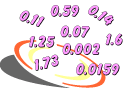Example 1: An electrician's apprentice earns \$7.58 per hour. If he earned \$316.99 last week, then about how many hours did he work?

Analysis: The phrase about how many indicates that we need to estimate. To solve this problem, we will estimate the quotient of these decimals. There are many strategies that we could use. We will use compatible numbers for this problem. The number 7.58 is close to 8 and the number 316.99 is close to 320.

Estimate: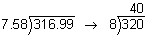In the example above, we changed the divisor 7.58 to 8 and the dividend 316.99 to 320. The numbers 8 and 320 are compatible since they make it easy to do mental arithmetic. Let's look at some more examples of estimating decimal quotients.

Example 2: Estimate the quotient: 4,189 ÷ 6.3

Analysis: Since 42 ÷ 6 = 7, we will round 4,189 to 4,200 and 6.3 to 6 .

Estimate: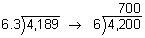Answer: The estimated quotient of 4,189 and 6.3 is 700.

In Example 2, each factor was changed to a compatible number. The numbers 4,200 and 6 are compatible since they make it easy to do mental arithmetic. When estimating decimal quotients, it is easiest to use compatible numbers. This is the strategy we will use throughout this lesson.

Example 3: Estimate the quotient: 3,041.9 ÷ 23

Analysis: Since 30 ÷ 2 = 15, we will round 3,041.9 to 3,000 and 23 to 20.

Estimate: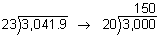Answer: The estimated quotient of 3,041.9 and 23 is 150.

Example 4: Estimate the quotient: 1,981.5 ÷ 401

Analysis: We will round 1,981.5 to 2,000 and 401 to 400.

Estimate:Answer: The estimated quotient of 1,981.5 and 401 is 5.

Now that we have learned how to estimate decimal quotients, we can look at some word problems.Example 5: If you bought 3 items for \$219.75, then about how much does each item cost?

Analysis: To solve this problem we need to estimate the quotient of \$219.75 and 3.

Estimate: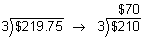Example 6: The Lachance family drove on a 1,587.2 km trip in 7.5 days. Estimate the average number of km driven per day.

Analysis: To solve this problem, we need to estimate the quotient of 1,587.2 and 7.5.

Estimate:Answer: The average number of km driven per day is about 200.Example 7: Nine jars of candy cost \$18.99. Derek estimated that he would have to pay \$2.00 for one jar. If the actual cost is \$2.11 for one jar, did he overestimate or underestimate? Explain your answer.

Answer: Derek underestimated because his estimated quotient of \$2.00 was lower than the actual cost of \$2.11.Example 8: Twenty-four blank DVDs cost \$70.80. Robin estimated that she would have to pay \$3.00 for one DVD. If the actual cost is \$2.95 for one DVD, did she overestimate or underestimate? Explain your answer.

Answer: Robin overestimated because her estimated quotient of \$3.00 was higher than the actual cost of \$2.95.

Example 9: Taylor divided 8,326 by 9.2 and got a quotient of 905. Use estimation to determine whether his answer is reasonable or unreasonable. Explain your answer.

Answer: Taylor's answer of 905 is reasonable since 8,100 ÷ 9 = 900.

Example 10: Nina divided 3,710.5 by 5.9 and got a quotient of 62.89. Use estimation to determine whether her answer is reasonable or unreasonable. Explain your answer.

Answer: Nina’s answer of 62.89 is unreasonable since 3,600 ÷ 6 = 600.

Summary: In this lesson, we learned how to estimate decimal quotients using compatible numbers. We also determined if an estimated quotient was an overestimate or an underestimate by comparing it with the actual answer. Lastly, we used estimation to determine if an answer was reasonable or unreasonable.

### Exercises

Directions: Read each question below. You may use paper and pencil to help you estimate. Select your answer by clicking on its button. Feedback to your answer is provided in the RESULTS BOX. If you make a mistake, choose a different button.

1.Charlotte was asked to estimate the quotient of 2,163 and 7.1. Which of the following compatible numbers did she use to get a reasonable estimate?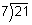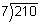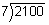None of the above.

RESULTS BOX:

2.Phil was asked to estimate the quotient of \$251.75 and 83. Which of the following compatible numbers did he use to get a reasonable estimate?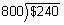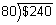None of the above.

RESULTS BOX:

 3.You are shopping in a video store and have \$49.50 to spend. DVDs cost \$3.25 each. Estimate the maximum number of DVDs you could buy. 3251.616None of the above. RESULTS BOX:
 4.Sarah divided 5,211.92 by 57.4 to find the quotient. Use estimation to determine which of the following answers is reasonable. 90.80908.09,080.None of the above. RESULTS BOX:
 5.There are 5 people in an elevator. Together they weigh 925.98 lbs. Use estimation to determine which of the following average weights is reasonable. 1,851.96 lbs.185.196 lbs.1.85196 lbs.None of the above. RESULTS BOX: